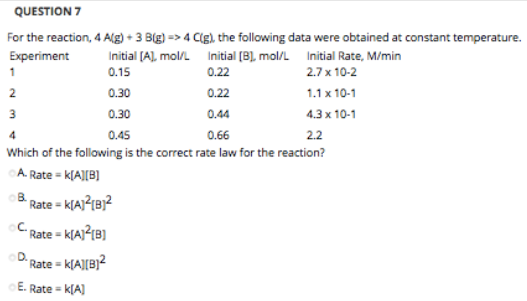# For the reaction, 4 A(g) + 3 B(g) => 4 C(g), the following data were obtained at constant temperature. Which of the following is the correct rate law for the reaction? a) Rate = k[A][B] b) Rate = k[A]^2[B] c) Rate = k[A]^2[B] d) Rate = k[A][B]^2 e) Rate = k[A]